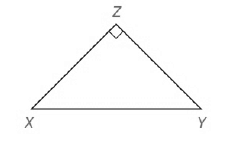Chapter 5.CT, Problem 10CTElementary Geometry For College St...

7th Edition
Alexander + 2 others
ISBN: 9781337614085

Solutions

Chapter
SectionElementary Geometry For College St...

7th Edition
Alexander + 2 others
ISBN: 9781337614085
Textbook Problem

In △ X Y Z , X Z - ≅ Y Z - and ∠ Z is a right angle.a) Find XY if XZ=10 in.b) Find XZ if X Y = 8 2 cm.To determine

a)

To find:

The value of XY.

Explanation

Given, In XYZ,

XZ¯YZ¯, therefore XZ¯=YZ¯

Z is a right angle.

XZ=10in.  and YZ=10in.

We have to find the hypotenuse XY.

By Pythagorean Theorem,

The square of the length of the hypotenuse of a right triangle is equal to the sum of the squares of the length of the legs

To determine

b)

To find:

The value of XZ.

Still sussing out bartleby?

Check out a sample textbook solution.

See a sample solution

The Solution to Your Study Problems

Bartleby provides explanations to thousands of textbook problems written by our experts, many with advanced degrees!

Get Started

In Exercises 516, evaluate the given quantity. log1616

Finite Mathematics and Applied Calculus (MindTap Course List)

In problems 15-26, evaluate each expression. 17.

Mathematical Applications for the Management, Life, and Social Sciences

Write in cylindrical coordinates, where E is the solid at the right.

Study Guide for Stewart's Multivariable Calculus, 8th

True or False: converges absolutely.

Study Guide for Stewart's Single Variable Calculus: Early Transcendentals, 8th